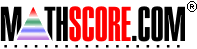Math Practice Online > free > lessons > Florida > 6th grade > Money Multiplication

## Money Multiplication

Multiply money by a single digit. You may need pencil and paper.

 Sample Problems for Money Multiplication Lesson for Money Multiplication

### This topic aligns to the following state standards

Grade 5: Num 2. Explains and demonstrates the multiplication of decimals to hundredths using concrete materials, drawings, story problems, symbols, and algorithms.
Grade 5: Num 1. Solves real-world problems involving addition, subtraction, multiplication, and division of whole numbers, and addition, subtraction, and multiplication of decimals, fractions, and mixed numbers using an appropriate method (for example, mental math, pencil and paper, calculator).

Copyright Accurate Learning Systems Corporation 2008.